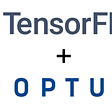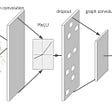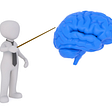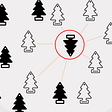# Principal Component Analysis by Example

Principal component analysis (PCA) is a dimension reduction algorithm. It transforms the data to a lower dimension, making it easy to visualize; for example, a human is a 3D object in space, a shadow of a human is a 2D representation of a human.

PCA utilizes Singular Value Decomposition (SVD). It is a factorization method used in linear algebra, it breakdown a matrix A into three matrices U, Σ, and VT.

• U: consists of column vectors
• Σ: consists of scalars
• VT: consists of row vectors

If you take the matrix multiplication of:

• The first column vector from U
• The first scalar from Σ
• The first row vector from VT

You get the first component, which has the maximum variance (most important data). The second component has the second maximum variance, and so on.

Let’s have an example:

Our data is the thumbs-up emoji.

First, we will load an image and convert it from RGB to grayscale (to make the example simple):

`with Image.open("thumbs-up.png") as im:   im = im.convert("L")`

Now, we convert the image to a NumPy array:

`A = np.asarray(im)plt.imshow(A)`

Finally, we compute the SVD:

`U, Sigma, VT = np.linalg.svd(A)`

If we plot the cumulative Σ, we can conclude the following:

`| Component(s) | Feature Importance ||--------------+--------------------||            1 |                30% ||            2 |                47% ||            5 |                64% ||           17 |                81% ||           51 |                98% |*Component(s) is also known as rank.*Feature Importance is cumulative.`

So, if we multiply U, Σ, and VT (given 51 components):

• 51 columns from U
• 51 scalars from S
• 51 rows from VT

We get a matrix that represents 98% of the original image, using the top 51 features.

Now, we plot the images using the values from the table:

`# c for components# @ is an operator for matrix multiplicationU[:,:c] @ Sigma[0:c,:c] @ VT[:c,:]`

As you can see, the first component represents 30% of the data, and the second data represents 17% of the data. If we pick these two components, we can represent 47% of the original data (it also implies that these two components have the most information about the data).

Image compression returns a good representation of the original image by picking the top features, with minimal loss in quality.

The complete notebook can be found here:

https://github.com/booletic/medium/tree/main/svd

--

--

--

## More from Mansoor Aldosari

https://github.com/booletic

Love podcasts or audiobooks? Learn on the go with our new app.

## Analysis of Tweets about the Joker (2019 film) in Python## Using Optuna to Optimize TensorFlow Hyperparameters## MACHINE LEARNING: AN OVERVIEW## Movie Search Engine using Tf-Idf## A brief introduction into data extraction from structured documents with Tesseract and Opencv## 5 Steps to Machine Learning with Google (Completely Free)## Understanding Natural Language## Neural Network## Mansoor Aldosari

https://github.com/booletic

## Linear Regression, A Quick, short but efficient note## K-Nearest Neighbors Classification## What is supervised learning and what are the different types of supervised learning algorithm?## A deep dive into Factor Analysis